# Test: Arithmetic And Geometric Progressions - 1

## 40 Questions MCQ Test Quantitative Aptitude for CA CPT | Test: Arithmetic And Geometric Progressions - 1

Description
Attempt Test: Arithmetic And Geometric Progressions - 1 | 40 questions in 40 minutes | Mock test for CA Foundation preparation | Free important questions MCQ to study Quantitative Aptitude for CA CPT for CA Foundation Exam | Download free PDF with solutions
QUESTION: 1

### Choose the most appropriate option ( a ), ( b ) , ( c ) or (d) The nth element of the sequence 1, 3, 5, 7,….…..Is

Solution:

Let a be the first term and d be the common difference.
Given series is in AP.

a = 1
d = 3 - 1 = 2

We know that nth term of an Ap an = a + (n - 1) * d

= 1 + (n - 1) * 2
= 1 + 2n - 2
= 2n - 1.

QUESTION: 2

### The nth element of the sequence –1, 2, –4, 8 ….. is

Solution: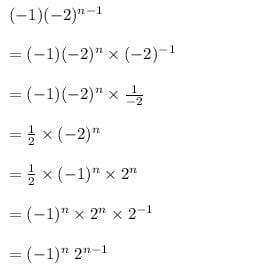QUESTION: 3

### The arithmetic mean between a and 10 is 30, the value of ‘a’ should be

Solution:
QUESTION: 4

–5, 25, –125 , 625, ….. can be written as

Solution:
QUESTION: 5

The first three terms of sequence when nth term tn is n2 – 2n are

Solution:
QUESTION: 6

Which term of the progression –1, –3, –5, …. Is –39

Solution:
QUESTION: 7

The value of x such that 8x + 4, 6x – 2, 2x + 7 will form an AP is

Solution:
QUESTION: 8

The mth term of an A. P. is n and nth term is m. The r th term of it is

Solution:
QUESTION: 9

An infinite GP has first term x and sum 5, then x belongs to

Solution:

We know that, the sum of infinite terms of GP is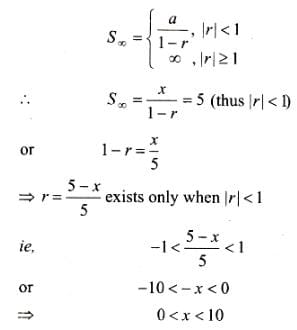QUESTION: 10

The nth term of the series whose sum to n terms is 5n2 + 2n is

Solution:

It is given that the sum of n terms is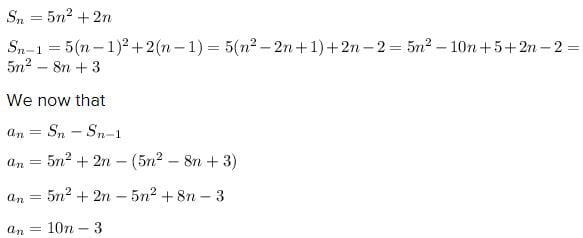QUESTION: 11

The 20th term of the progression 1, 4, 7, 10.................is

Solution:
QUESTION: 12

The last term of the series 5, 7, 9,….. to 21 terms is

Solution:
QUESTION: 13

The last term of the A.P. 0.6, 1.2, 1.8,… to 13 terms is

Solution:
QUESTION: 14

The sum of the series 9, 5, 1,…. to 100 terms is

Solution:
QUESTION: 15

The two arithmetic means between –6 and 14 is

Solution:

Let the terms be – 6, a, b 14

a = – 6

T4 = a + 3d = 14

– 6 + 3d = 14

3d = 20

d = 20/3

a = – 6 + 20/3 = 2/3

b = 2/3 + 20/3 = 22/3

QUESTION: 16

The sum of three integers in AP is 15 and their product is 80. The integers are

Solution:

let a - d , a , a + d are three terms of an AP

according to the problem given,

sum of the terms = 15

a - d + a + a + d = 15

3a = 15

a = 15 / 3

a = 5

product = 80

( a - d ) a ( a + d ) = 80

( a² - d² ) a = 80

( 5² - d² ) 5 = 80

25 - d² = 80 /5

25 - d² = 16

- d² = - 9

d² = 3²

d = ± 3

Therefore,

a = 5 , d = ±3

required 3 terms are

a - d = 5 - 3 = 2

a = 5

a+ d = 5 + 3 = 8

( 2 , 5 , 8 ) or ( 8 , 5 , 2 )

QUESTION: 17

The sum of n terms of an AP is 3n2 + 5n. A.P. is

Solution:

The sum of n terms of an A.P. = 3n2+5n

then sum of n-1 terms= 3(n-1)2+5(n-1)

So nth term of A.P will be Tn=3n2+5n- 3(n-1)2-5(n-1)= 3n2+5n-3n2-3+6n-5n+5=6n+2

in this way by putinng values of n= 1,2,3,4,5,6,7,_______n-1, n

we will get the AP as 8,14,20,26,32,38,44,____

QUESTION: 18

The number of numbers between 74 and 25556 divisible by 5 is

Solution:
QUESTION: 19

The pth term of an AP is (3p – 1)/6. The sum of the first n terms of the AP is

Solution:
QUESTION: 20

The arithmetic mean between 33 and 77 is

Solution:
QUESTION: 21

The 4 arithmetic means between –2 and 23 are

Solution:

a=-2 n=6

given : t6= 23

23= a+5d

23= -2+5d

d= 25/5

d = 5

t2= a+d= -2+5=3

t3= 8

t4= 13

t5= 18

QUESTION: 22

The first term of an A.P is 14 and the sums of the first five terms and the first ten terms are equal is magnitude but opposite in sign. The 3rd term of the AP is

Solution:
QUESTION: 23

The sum of a certain number of terms of an AP series –8, –6, –4, …… is 52. The number of terms is

Solution:
QUESTION: 24

The 1st and the last term of an AP are –4 and 146. The sum of the terms is 7171. The number of terms is

Solution:
QUESTION: 25

The sum of the series 3 ½ + 7 + 10 ½ + 14 + …. To 17 terms is

Solution:
QUESTION: 26

The 7th term of the series 6, 12, 24,……is

Solution:
QUESTION: 27

t8 of the series 6, 12, 24,…is

Solution:
QUESTION: 28

t12 of the series –128, 64, –32, ….is

Solution:
QUESTION: 29

The 4th term of the series 0.04, 0.2, 1, … is

Solution:
QUESTION: 30

The last term of the series 1, 2, 4,…. to 10 terms is

Solution:
QUESTION: 31

The last term of the series 1, –3, 9, –27 up to 7 terms is

Solution:
QUESTION: 32

The last term of the series x2, x, 1, …. to 31 terms is

Solution:
QUESTION: 33

The sum of the series –2, 6, –18, …. To 7 terms is

Solution:
QUESTION: 34

The sum of the series 24, 3, 8, 1, 2, 7,… to 8 terms is

Solution:

Sum of series 24,3,8,1,2,7..to 8th term is:
Take the 1st and 2nd terms, 24,3, put them together, 243 = 3^5.
Take the 3rd and 4th terms, 8,1, put them together, 81 = 3^4.
Take the 5th and 6th terms, 2,7, put them together, 27 = 3^3.
Take the 7th and 8th terms, 0,9, put them together, 09 = 3^2.
Sum of 8 terms = 24+3+8+1+2+7+0+9 = 54

QUESTION: 35

The sum of the series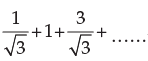to 18 terms is

Solution:
QUESTION: 36

The second term of a G P is 24 and the fifth term is 81. The series is

Solution:
QUESTION: 37

The sum of 3 numbers of a G P is 39 and their product is 729. The numbers are

Solution:
QUESTION: 38

In a G. P, the product of the first three terms 27/8. The middle term is

Solution:
QUESTION: 39

If you save 1 paise today, 2 paise the next day 4 paise the succeeding day and so on, then your total savings in two weeks will be

Solution:

If we save 1 paise today,2 paise the next day,4 pause the succeeding day and so on then you total savings in two weeks the sequence should be Geometric progression .

1, ,2,4, 8, 16, .....,14 terms

First term (a) = 1,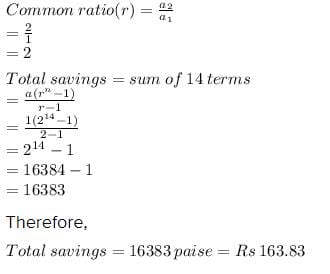QUESTION: 40

Sum of n terms of the series 4 + 44 + 444 + … is

Solution:

S=4+44+444+-------n terms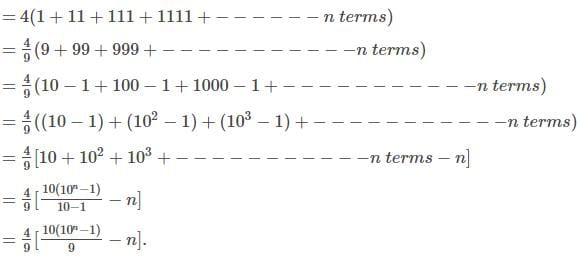Use Code STAYHOME200 and get INR 200 additional OFF Use Coupon Code